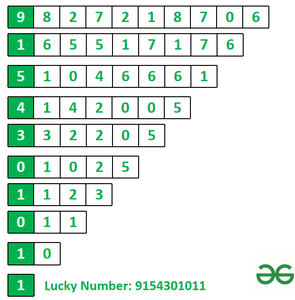# Find the Mobile Number formed using first digits of arrays of absolute differences of consecutive numbers

• Difficulty Level : Medium
• Last Updated : 02 Mar, 2022

Given a String ph[], the task is to find a new number for the user, based on following conditions:

1. The new number will also start from the same digit as of original number.
2. The digits of the new number will be the first digits of a series of arrays of absolute differences of the consecutive elements.

Examples:

Input: ph = “9827218706”
Output: 9154301011
Explanation:Input: ph =”9647253846″
Output: 9310100011

Approach: Consider the following steps to solve this problem:

1. Convert every character from the string into integer and store it into the array ph1[] using list comprehension.
2. Declare an empty string ph2.
3. Convert first element of array ph1[ ] into a string and add it to ph2.
4. Using List comprehension create an array by storing the absolute difference of consecutive elements.
5. Assign this array to ph1.
6. Repeat step 3-5, ten times as the phone number have ten digits.

Below is the implementation of the above approach.

## Python3

 `# Function to find lucky phone number``def` `phone(ph, n):` `    ``# Converting char to int and storing into array.``    ``ph1 ``=` `[``int``(i) ``for` `i ``in` `ph]` `    ``# Empty string to store lucky number.``    ``ph2 ``=` `""` `    ``# Loop for performing action``    ``# and adding digit to ph2.``    ``for` `_ ``in` `range``(n):` `        ``# Convert first element into``        ``# string and adding to ph2.``        ``ph2 ``+``=` `str``(ph1[``0``])` `        ``# Creating new ph1 by subtracting``        ``# consecutive element.``        ``ph1 ``=` `[``abs``(ph1[j]``-``ph1[j ``+` `1``]) \``              ``for` `j ``in` `range``(``len``(ph1)``-``1``)]` `    ``# Return lucky number ph2``    ``return` `ph2`  `# Original number``ph ``=` `"9827218706"` `# Calling phone function.``num ``=` `phone(ph, ``len``(ph))` `# Print the lucky number``print``(num)`

## Javascript

 ``
Output
`9154301011`

Time Complexity: O(N*N)
Auxiliary Space: O(N)

Efficient Approach: In this approach, no extra space is required for storing elements in the array. First, declare an empty string ph2 in which lucky number will be stored, now create a for loop in which the first character of the string will be added to ph2 and again another for loop to find the absolute difference of consecutive element. Now the string of absolute difference will be assigned to ph1 which is the original number and the same steps will be followed. Follow the steps below to solve the problem:

Below is the implementation of the above approach.

## C++

 `// C++ code for the above approach``#include ``using` `namespace` `std;` `// Function to find lucky number.``string phone(string ph, ``int` `n)``{` `    ``// ph2 is empty string to store lucky number.``    ``string ph2 = ``""``;` `    ``// For loop for finding lucky number``    ``for` `(``int` `i = 0; i < ph.length(); i++) {` `        ``// Add first element of ph to ph2``        ``ph2 += ph;` `        ``// S for storing the difference``        ``string S = ``""``;` `        ``// Loop to calculate the absolute difference``        ``for` `(``int` `j = 0; j < ph.length(); j++) {``            ``int` `x = ``abs``(``int``(ph[j]) - ``int``(ph[j + 1]));``            ``S += x + ``'0'``;``        ``}` `        ``// Assigning S to ph.``        ``ph = S;``    ``}` `    ``// Return the lucky number``    ``return` `ph2;``}` `// Driver Code``int` `main()``{` `    ``// Original number``    ``string ph = ``"9827218706"``;` `    ``// Call phone function``    ``string num = phone(ph, ph.length());` `    ``// Printing lucky number``    ``cout << (num);``}` `// This code is contributed by Potta Lokesh`

## Java

 `// Java program for the above approach``import` `java.util.*;`` ``class` `GFG {` `  ``// Function to find lucky number.``  ``static` `String phone(String ph, ``int` `n)``  ``{` `    ``// ph2 is empty string to store lucky number.``    ``String ph2 = ``""``;` `    ``// For loop for finding lucky number``    ``for` `(``int` `i = ``0``; i < ph.length(); i++)``    ``{` `      ``// Add first element of ph to ph2``      ``ph2 += ph.charAt(``0``);` `      ``// S for storing the difference``      ``String S = ``""``;` `      ``// Loop to calculate the absolute difference``      ``for` `(``int` `j = ``0``; j < ph.length()-``1``; j++)``      ``{``        ``int` `x = Math.abs(ph.charAt(j) - ph.charAt(j+``1``));``        ``S += (``char``)(x + ``'0'``);``      ``}` `      ``// Assigning S to ph.``      ``ph = S;``    ``}` `    ``// Return the lucky number``    ``return` `ph2;``    ` `  ``}` `  ``// Driver Code``  ``public` `static` `void` `main(String args[])``  ``{``    ``// Original number``    ``String ph = ``"9827218706"``;` `    ``// Call phone function``    ``String num = phone(ph, ph.length());` `    ``// Printing lucky number``    ``System.out.println(num);``  ``}``}` `// This code is contributed by avijitmondal1998`

## Python3

 `# Function to find lucky number.``def` `phone(ph, n):` `    ``# ph2 is empty string to store lucky number.``    ``ph2 ``=` `""` `    ``# For loop for finding lucky number``    ``for` `i ``in` `range``(``len``(ph)):` `        ``# Add first element of ph to ph2``        ``ph2 ``+``=` `ph[``0``]` `        ``# S for storing the difference``        ``S ``=` `""` `        ``# Loop to calculate the absolute difference``        ``for` `j ``in` `range``(``len``(ph)``-``1``):``            ``x ``=` `abs``(``int``(ph[j])``-``int``(ph[j ``+` `1``]))``            ``S ``+``=` `str``(x)` `        ``# Assigning S to ph.``        ``ph ``=` `S` `    ``# Return the lucky number``    ``return` `ph2`  `# Original number``ph ``=` `"9827218706"` `# Call phone function``num ``=` `phone(ph, ``len``(ph))` `# Printing lucky number``print``(num)`

## C#

 `// C# code for the above approach``using` `System;``class` `GFG``{` `  ``// Function to find lucky number.``  ``static` `string` `phone(``string` `ph, ``int` `n)``  ``{` `    ``// ph2 is empty string to store lucky number.``    ``string` `ph2 = ``""``;` `    ``// For loop for finding lucky number``    ``for` `(``int` `i = 0; i < ph.Length; i++)``    ``{` `      ``// Add first element of ph to ph2``      ``ph2 += ph;` `      ``// S for storing the difference``      ``string` `S = ``""``;` `      ``// Loop to calculate the absolute difference``      ``for` `(``int` `j = 0; j < ph.Length; j++)``      ``{``        ``int` `x = Math.Abs(ph[j] - ph[j + 1]);``        ``S += x + ``'0'``;``      ``}` `      ``// Assigning S to ph.``      ``ph = S;``    ``}` `    ``// Return the lucky number``    ``return` `ph2;``  ``}` `  ``// Driver Code``  ``public` `static` `void` `Main()``  ``{` `    ``// Original number``    ``string` `ph = ``"9827218706"``;` `    ``// Call phone function``    ``string` `num = phone(ph, ph.Length);` `    ``// Printing lucky number``    ``Console.WriteLine (num);``  ``}``}` `// This code is contributed by ukasp.`

## Javascript

 ``
Output
`9154301011`

Time Complexity: O(N*N)
Auxiliary Space: O(N)

My Personal Notes arrow_drop_up